# My Notes: Arrays (CS50)

### 4/7/2020

Today, it's more about going underneath the hood on programming. Looking at principles of programming, it won't feel magical and we will understand how things work.

Some basic code below from last week -

``````    #include <cs50.h>
#include <stdio.h>

int main(void)
{
string name = get_string("Whats your name?\n");
printf("HI\n");
}
``````

What is main()? It is the main function of the program

printf() is also a function, can take in one input. it is part of stdio library.

stdio is a library, someone elses code. it is a header file in C. It has the prototype of printf().

\n is new line

``````    clang -o hello hello.c -lcs50
./hello

``````

Above are commands in the terminal. When you compile your program with clang, it creates a.out file with machine code.

clang -o is a command line argument to rename the output file. lcs is to link in the cs50 library. Using the 'make' command simplifies these commands.

Running make or clang commands, there are 4 steps.

1) preprocessing

2) compiling

3) assembling

Preprocessing

``````    ...
string get_string(string prompt);
int printf(string format, ...);
...

int main(void)
{
string name = get_string("Whats your name?\n");
print("hello, %s\n", name);
}

``````

During preprocessing, when you run clang (or run make command), the '#' lines (library lines), clang takes the relevant lines from the library and copy and pastes into your code. In the above code, we replaced the #include lines and put the functions which are borrowed from those libraries.

Compiling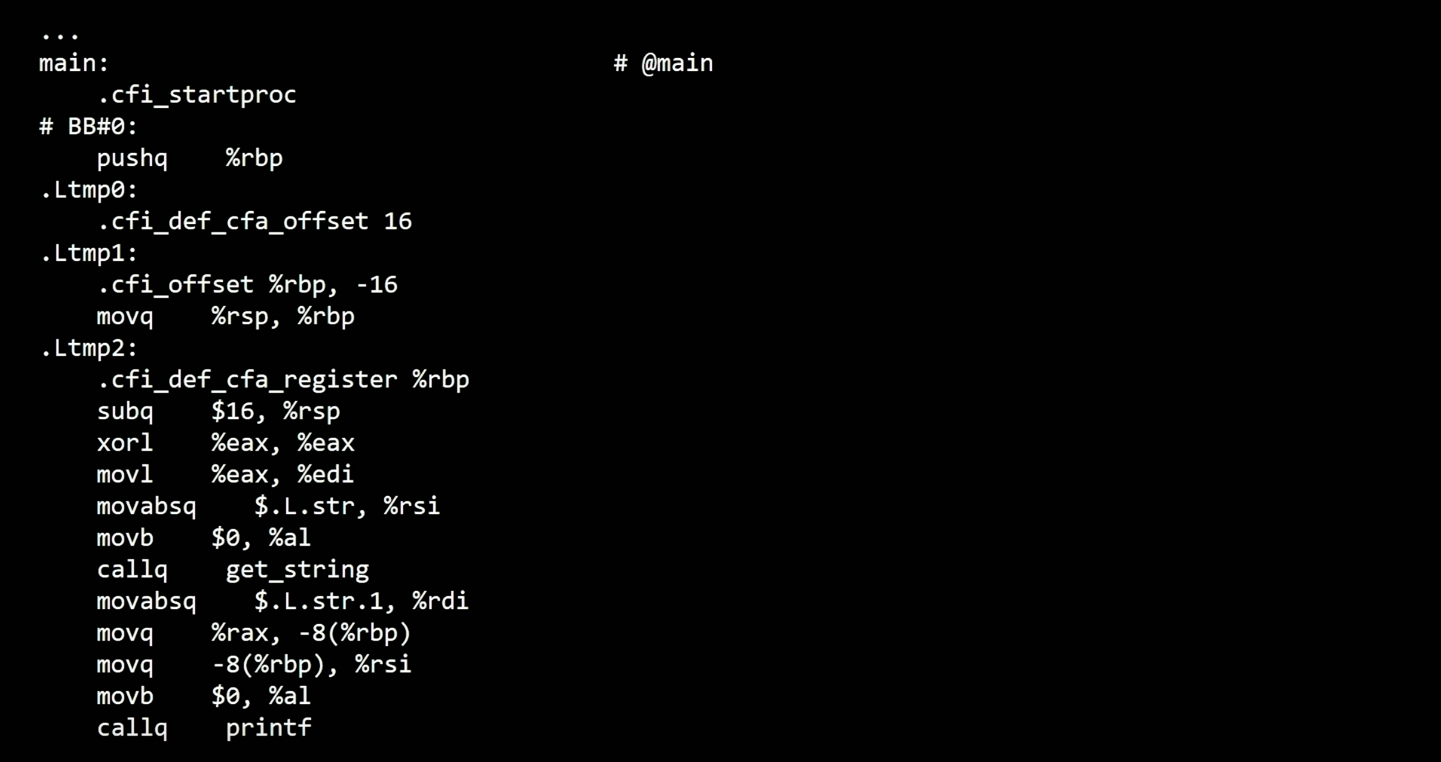C code changes via clang to assembley code. Some words may look familiar. This is closer to what CPU will understand. There are some instructions for the CPU in here (pushq, movq,subq,)...these are things to move around in memory, etc. Its not for us, clang does this for us.

Assembling

Another thing clang does. It makes the assembly code 0s and 1s to machine code.

All the machine code (from printf, get string, etc.) links up into one file, a.out.

--

Debugging

Bugs are mistakes in programs.

We are now using an IDE instead of the Sandbox from last week. An IDE is an integrated development environment. Essentially, there are a few more tools included in this environment, as opposed to a more basic sandbox. The IDE includes a debugger, which is a very useful tool. A debugger will run your code step by step, see where it goes wrong. There are debugger features in modern browsers (Firefox, Chrome, etc.). You can run through the code step by step, seeing what is changing, local variables (and their values), functions invoked, etc.

If there is a problem with the syntax, most likely the code won't run and you will recieve an error message in the terminal.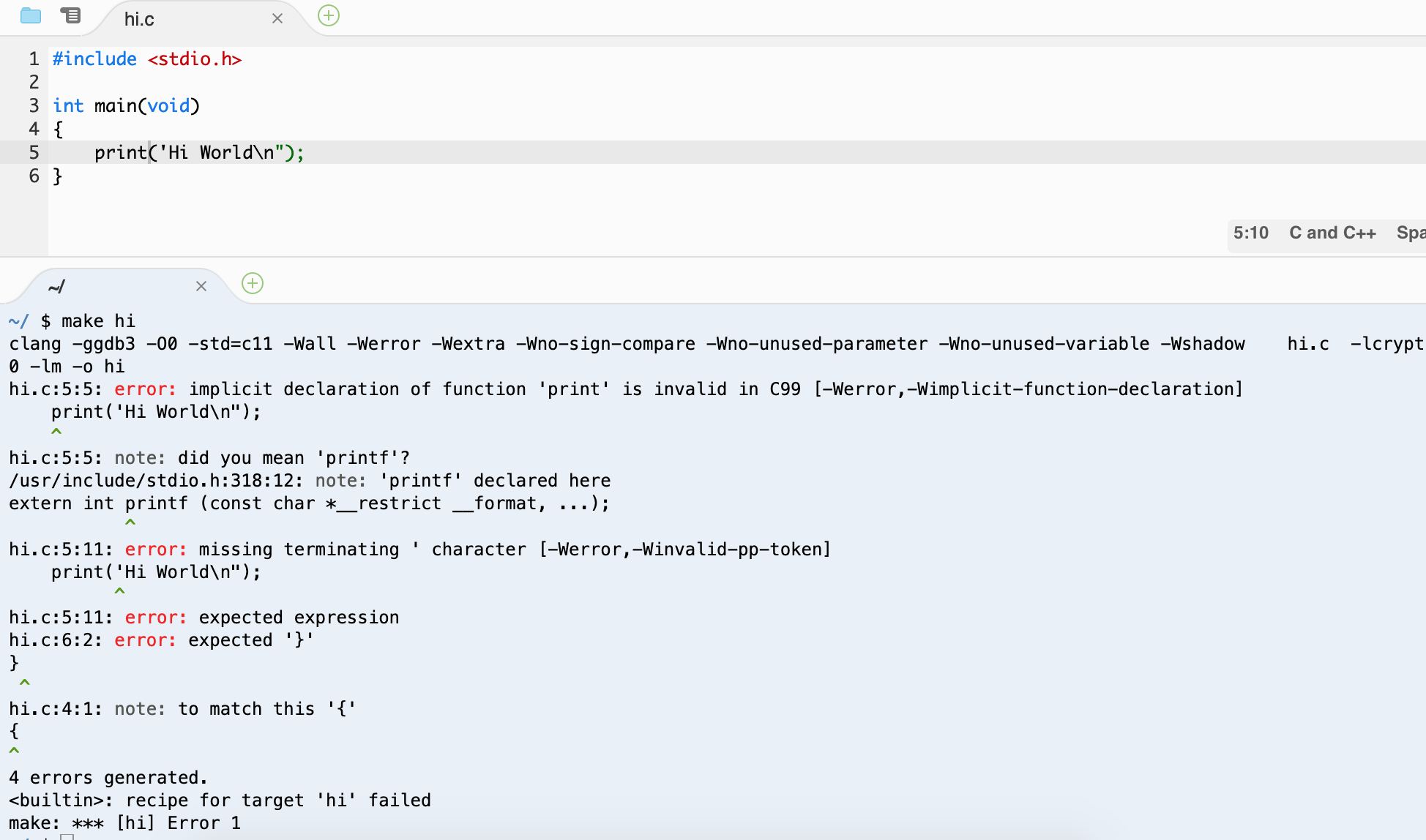CS50 IDE has a few built in commands which help you debug, instead of having to disect those overwhelming error messages.

(Just prefix your commands with these)

• help50 - Help simplify the error message, something much more readable.
• check50 - Runs tests on your program to verify that certain things are done or written correctly
• style50 - Makes sure syntax is proper, the computer can still run it as it is valid code but it will suggest styling things to make the code more readable (like indenting, etc.)
• debug50

Most programming languages will have 'style guides' -- how to make your code more readable, this is opinionated though.

Besides syntax problems, you may run into logical problems. This is where printf (or console.log in JS) can be very helpful. Program executes w/o errors so you can see at least what is going on.

How else to debug? Taking a break from the code always helps, refreshs the mind.

Rubberduck debugging - talk it out, line by line, explaining what is going on in each line of code. It will help you think, and hopefully catch where the program is faltering.

Design

Subjective...the process of writing well designed software. Not just solve problems, but solve the problem well. When involving data and users, must design programs efficiently as it may take up large resources (memory, time).

How to write better designed code? Lets explore...

Storing Data

Data types take up certain bytes. Computers have RAM (random access memory). RAM is where information is stored while the programs are running. There is a finite number of bytes in RAM...think of each data type, how many bytes they're taking up.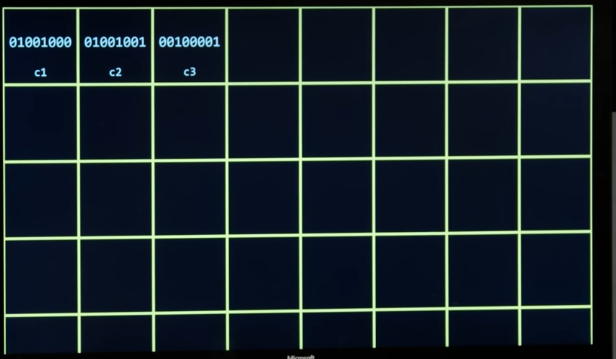How is the computer storing the information?

``````    #include <stdio.h>

int main(void)
{
char c1 = 'H';
char c2 = 'I';
char c3 = '!';
printf("%c %c %c\n", c1 c2 c3);
}
``````

**Char take single quotes, not double

Casting: the act of converting one data type to another

``````    #include <stdio.h>

int main(void)
{
char c1 = 'H';
char c2 = 'I';
char c3 = '!';
printf("%i %i %i\n", (int) c1, (int) c2, (int) c3);
}
``````

Above will print char's numbers, you get the ASCII values of "H I !"

Arrays

The below program could be designed a little better. If you know you have or will have closely related values, consider using an array.

``````    #include <stdio.h>

int main(void)
{
int score1 = 72;
int score2 = 73;
int score3 = 33;

printf("Average: %i\n", (score1 + score2 + score3) / 3);
}
``````

Could we store scores together?

An array is a list of values, all the same data type, in one variable. We could put all 3 scores in an array. There are a few different ways to initialize an array. Arrays are zero indexed, so start at 0, not 1.

``````    int scores;
scores = 72;
scores = 73;
scores = 33;
``````

There is a more readable way to write out the array. Code still seems a little verbose. The above code violates the DRY (Don't Repeat Yourself) principle. It can be rewritten more efficiently (we can also make it more dynamic and less hardcoded).

You can use a constant (const) to hardcode a value if you know it won't change (JS has introduced const recently).

``````    const int N = 3;
``````

Global variables, try to avoid, unless you're going to use a const.

``````    #include <stdio.h>

const int N = 3 /* Global variable, as declared outside the function*/

int main(void)
{
int scores[N];
scores = 72;
scores = 73;
scores = 33;
printf("Average: %i\n", (score + score + score) / N);
}
``````

We can make this more dynamic by asking the user for input, then store the input and produce the output (output being the average). We can also use a float for a more fair average (as we've just been using integers). Here is the program, taking in inputs and using a loop.

``````    #include <stdio.h>
#include <cs50.h>

float average(int length, int array[]); /* Using prototype here so it is still defined */

int main(void)
{
int n = get_int("Number of scores: ");

int scores[n];

for (int i = 0; i < n; i++)
{
scores[i] = get_int("Score: ");
}

printf("Average: %.1f\n", average(n, scores)); /* Floating point here, .1 is for one floating digit only, aka rounding */
}

float average(int length, int array[])
{
int sum = 0;
for (int i = 0; i < length; i++)
{
sum += array[i];
}
return (float) sum / (float) length; /* Using casting here */
}
``````

Back to Memory...and Data Types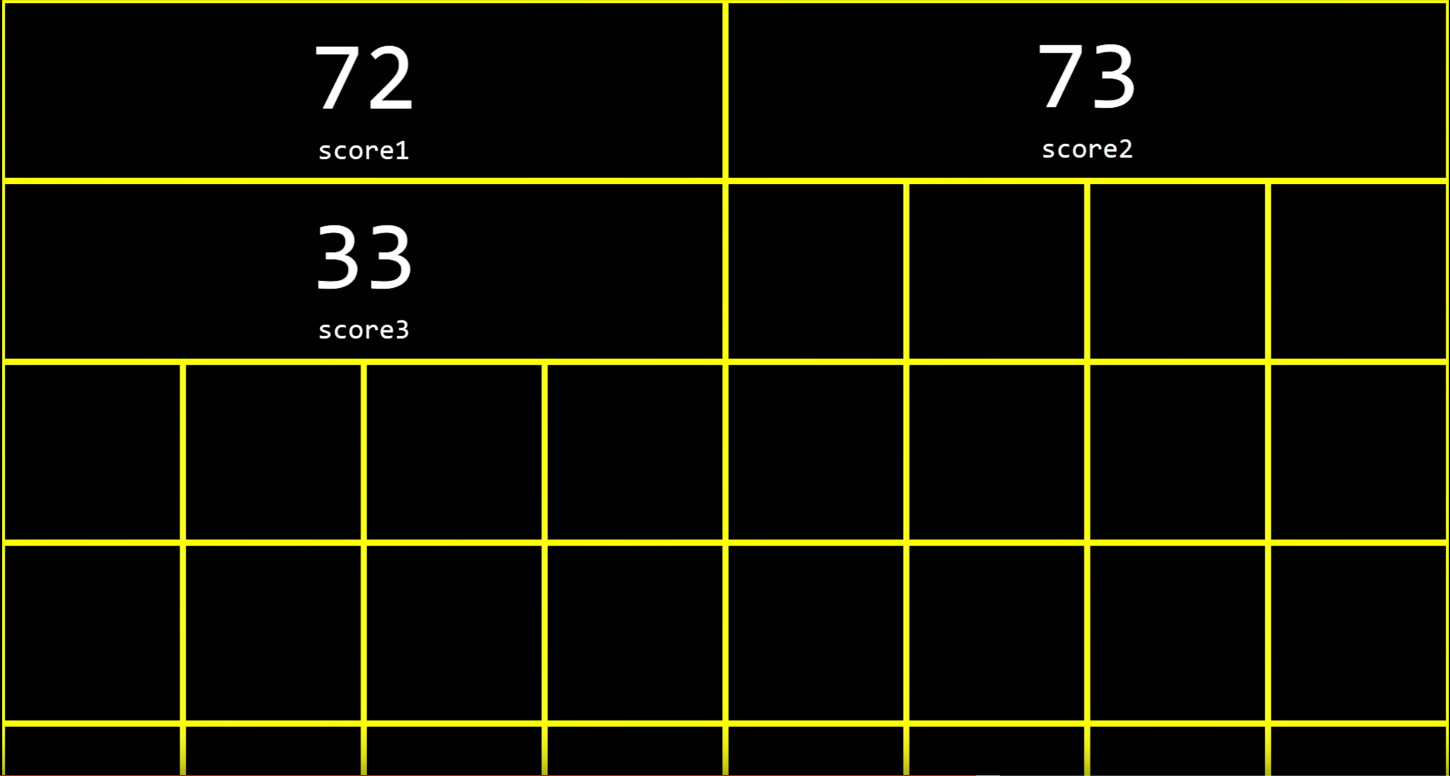``````    int score1 = 72;
int score2 = 73;
int score3 = 33;

``````

Stored in memory like below. ints are 4 bytes

A string is just an array of characters.

``````    string s = "HI!";
s = 'H';
s = 'I';
s = '!';
``````

Strings can't have a pre-associated length as they vary in bytes.

How do you know when the string ends? There is a null terminating character. It is eight straight 0s to let the computer know the end of the string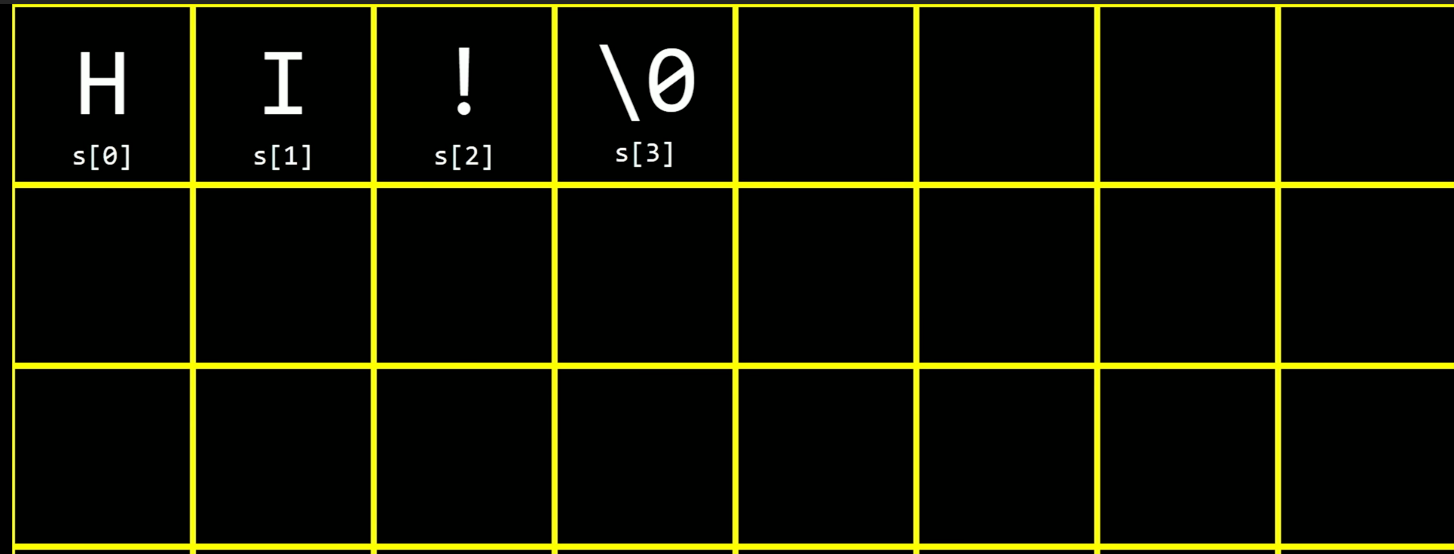String with a length 3 takes up 4 bytes (as seen above).

``````    int main(void)
{
strings names;
names = "EMMA";
names = "RODRIGO";
names = "BRIAN";
names = "DAVID";

printf("%s\n", names); /* Printing "EMMA" */
}
``````

Here we see how the strings from the names array are stored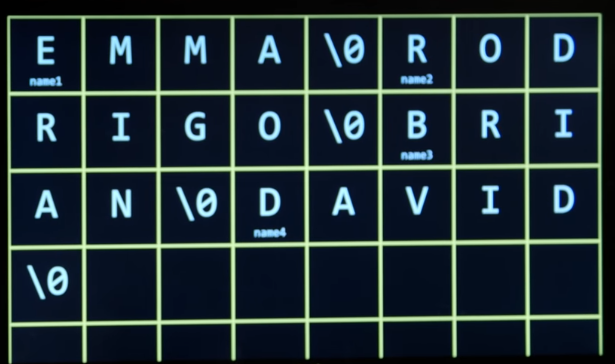Any character from any string in the array is accessible, using the double bracket notation

Looking more at the standard code we've been using, line by line.

``````#include <stdio.h>
``````

Imports prototypes from other libraries (other functions or helpers that others have written already). So we can use in our code.

``````int main(void)
``````

Void..what is it? What is int? In C, you don't need to just write void in, you can use...

``````int main(int argc, string argv[])
``````

The above takes in two arguments. One is an integer, second is an array of strings. To specify, 'argc' is argument count and 'argv' is argument vector. We can implement features like clang has.

``````    #include <cs50.h>
#include <stdio.h>

int main(int argc, string argv[])
{
if (argc == 2) /* User has typed two words at the prompt */
{
printf("hello, %s\n", argv);
}
else /* User has not written anything at command prompt */
{
printf("hello, world\n");
}
}
``````

If above is named name.c file, we run --

``````    make name                    /* CS50 outputs name.c */
./name                       /*  "hello, world" */
./name Bob               /*  "hello, Bob"  */
``````

In the second line, there are no arguments provided, so defaults to the else statement.

Why does main have a return value of int? By default, main returns 0. In computers, 0 means all is okay. Main can return non zero values.

--